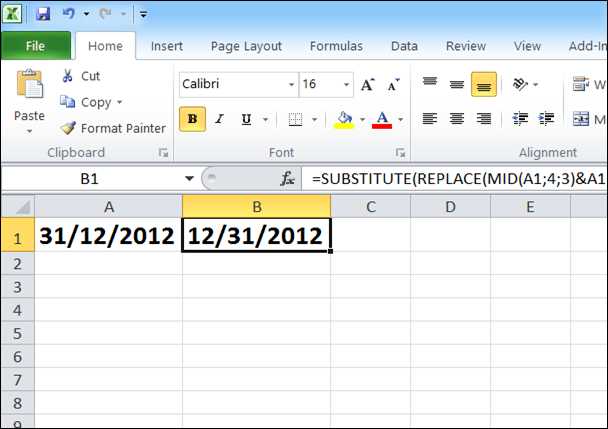Thursday, July 10, 2014

Convert Date String in Excel 2010

I got series of data ini Microsoft Excel 2010 that have date data, but the date is in the text format. I want to convert the text format from dd/mm/yyyy to mm/dd/yyyy because I need to insert them into database that require date format in sql is in mm/dd/yyyy format.Then i put all date data in column A and put his formula in an empty column:

=SUBSTITUTE(REPLACE(MID(A1;4;3)&A1;7;3;"");"/";"/")+0

This will convert date in the text format in cell A1 from 31/12/2012 to 12/31/2012 and I can format the result of formula as date. This will not work if the data is already in the date format. In that case, no formula needed, just right click on the cells and format the cells as date.

If you're in the US and have a worksheet with dates in UK format then some of these will probably be recognised as dates, but the wrong date, for example, 3th April becomes 4th March and some others, where the day is greater than 12, are not recognised as dates at all, so excel treats these as text.

This formula will work if you just need text replacement. You just need to remove “+0” in the MS Excel formula:

=SUBSTITUTE(REPLACE(MID(A1;4;3)&A1;7;3;"");"/";"/")

If you want to replace date in “Europan” format dd.mm.yyyy to mm/dd/yyyy, just change the MS Excel formula to:

=SUBSTITUTE(REPLACE(MID(A1;4;3)&A1;7;3;"");".";"/")

If the formula not working, some excel version and with different locale configuration need to change the semicolon to comma, for example:

=SUBSTITUTE(REPLACE(MID(A1,4,3)&A1,7,3,""),".","/")